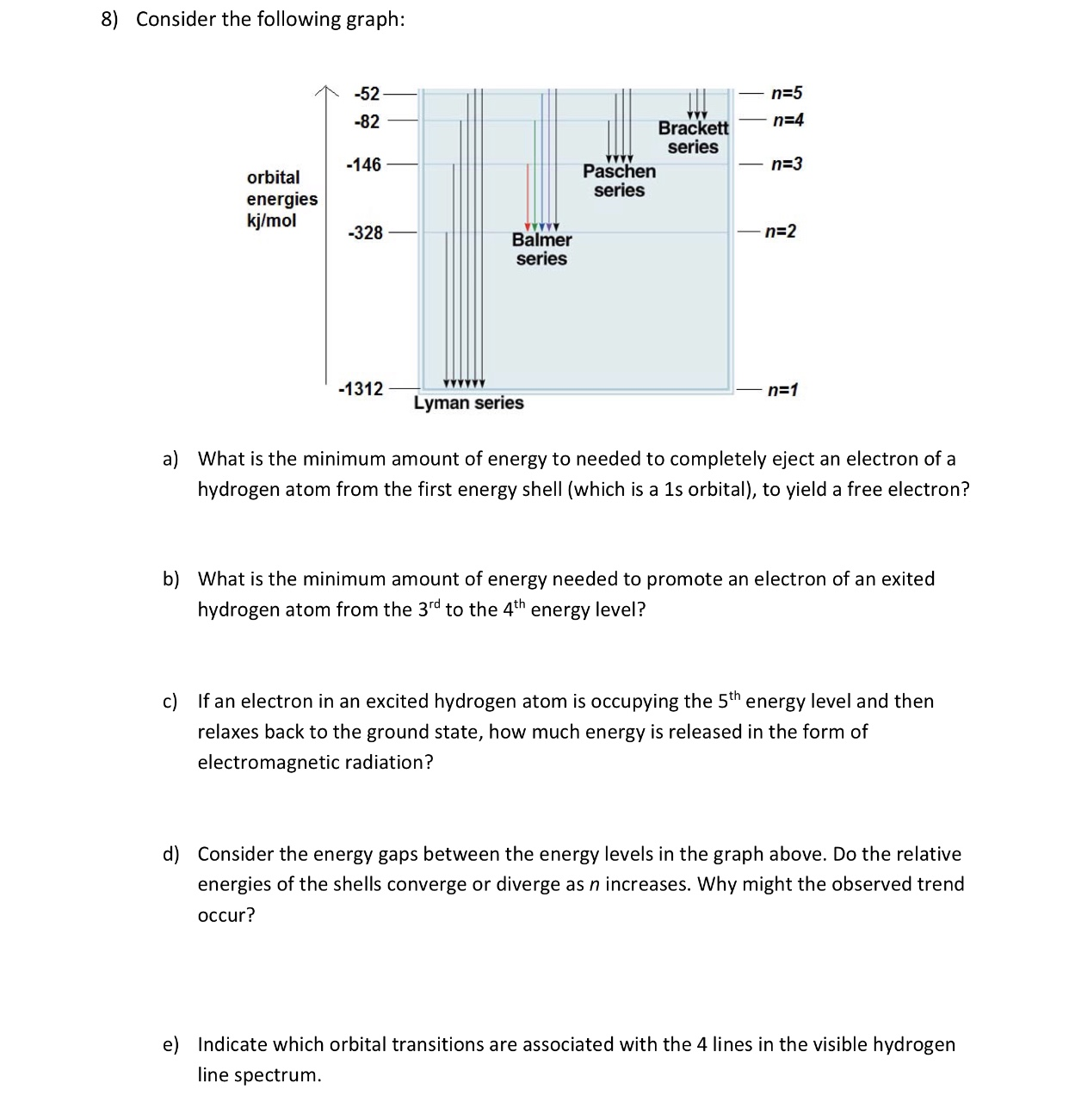# 8) Consider the following graph: n-5 n-4 -52 -82 -146 Brackett series n 3 Paschen series orbital energies kj/mol 328 n-2 Balmer Series -1312 Lyman series a) What is the minimum amount of energy to needed to completely eject an electron of a hydrogen atom from the first energy shell (which is a 1s orbital), to yield a free electron? b) What is the minimum amount of energy needed to promote an electron of an exited hydrogen atom from the 3rd to the 4th energy level? If an electron in an excited hydrogen atom is occupying the 5th energy level and then relaxes back to the ground state, how much energy is released in the form of electromagnetic radiation? c) d) Consider the energy gaps between the energy levels in the graph above. Do the relative energies of the shells converge or diverge as n increases. Why might the observed trend occur? e) Indicate which orbital transitions are associated with the 4 lines in the visible hydrogen line spectrum

Questionhelp_outlineImage Transcriptionclose8) Consider the following graph: n-5 n-4 -52 -82 -146 Brackett series n 3 Paschen series orbital energies kj/mol 328 n-2 Balmer Series -1312 Lyman series a) What is the minimum amount of energy to needed to completely eject an electron of a hydrogen atom from the first energy shell (which is a 1s orbital), to yield a free electron? b) What is the minimum amount of energy needed to promote an electron of an exited hydrogen atom from the 3rd to the 4th energy level? If an electron in an excited hydrogen atom is occupying the 5th energy level and then relaxes back to the ground state, how much energy is released in the form of electromagnetic radiation? c) d) Consider the energy gaps between the energy levels in the graph above. Do the relative energies of the shells converge or diverge as n increases. Why might the observed trend occur? e) Indicate which orbital transitions are associated with the 4 lines in the visible hydrogen line spectrum fullscreen

1 Rating

### Want to see the step-by-step answer?